Rocket Equations

Equations for model rocketeers - how to accurately predict speed and altitude for your rocket from weight, diameter, motor thrust and impulse.

Related Pages

• Rocket Equations Quick Reference: Fast and pretty one page summary of the equations on this page.
• Multistage Rocket Equations: extension of the equations on this page to the problem of finding speed and altitude for multistage rockets.
• Rocket Simulations: explanation of basic physics and how you can program or spreadsheet your own simulation.
•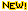Rocket Simulations in 2D: explanation how to include launch angle in your calculations to find the down-range distance your rocket will go.
• Applied Physics: some other handy equations for estimating launch velocity and proper motor size for your rocket.
• Parachute Descent Calculations: How do you determine the right size for your parachute? This page shows you how to find chute size and descent velocity.
• Thrust and Mass Studies: I plotted some curves using these equations to show how changing a rocket's mass or changing motor thrust affects the resulting flight altitude.
• Barrowman Equations: determine the stability of your rocket by calculating the center of pressure - with spreadsheet example.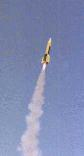How High Will My Rocket Go?

• At first the equations look rough and tough but they're creampuffs. They look bad because web page writing (html) just isn't made for writing equations (they look better here). If you could find this page you can handle the calculations.
• These equations include the effect of wind resistance and therefore they're accurate. Drag effects will have a huge effect on calculated results (factor of two to four!) but most authors view them as "beyond the scope of the text" and don't tell you how to include them. Formulas with wind resistance are so rare that I had to derive them myself (see below).
• This is a problem usually solved by running computer simulations. With this page you can do it yourself by hand - COOL, HUH? Plug the equations into a spreadsheet and compare performance of models out of the catalogues, or see how different motors will affect the speed, altitude, or coast time of your rocket. You can do what I do - plug the equations into your programmable calculator and estimate altitudes and coast times in the field, as you're prepping your rockets.
• Everything here is converted into metric units. The resulting velocity is computed in meters/second, and altitude is in meters. Multiply by 3.281 to convert to feet/second or altitude in feet, multiply meters/second by 2.237 to get miles per hour.
• Note that there are two phases to consider for the calculation (with or without drag): the "boost phase" and the "coast phase".
• Boost phase is while the rocket motor is burning,
• Coast phase is the time from engine burn-out to peak altitude.

The Equations

There are three basic equations to find the peak altitude for your rocket (they are also summarized here):

• Max velocity v, the velocity at burnout = q*[1-exp(-x*t)] / [1+exp(-x*t)]
• Altitude reached at the end of boost = [-M / (2*k)]*ln([T - M*g - k*v^2] / [T - M*g])
• Additional height achieved during coast = [+M / (2*k)]*ln([M*g + k*v^2] / [M*g])

All the weird terms in these equations are explained in the following section on the method for using the equations.

The Method

1. Compute Some Useful Terms
• Find the mass M of your rocket in kilograms (kg):
M = (weight in ounces)/16/2.2
• Find the area A of your rocket in square meters (m^2):
A = pi*(0.5*(diameter in inches/12)*0.3048)^2 = pi*r^2
where r is the radius in meters
• Note that the wind resistance force = 0.5 * rho*Cd*A * v^2, where
rho is density of air = 1.2 kg/m^3
Cd is the drag coefficient of your rocket which is around 0.75 for a model rocket - for a really great NAR report on Cd values click here
v is the velocity of the rocket. You don't calculate this drag force, though, since you don't know what "v" is yet. What you do need is to lump the wind resistance factors into one coefficient k:
k = 0.5*rho*Cd*A = 0.5*1.2*0.75*A
• Find the impulse I and thrust T (big T) of the engine for your rocket from the engine designator, for an explanation you can reference NAR's Rocket Motor Coding, the NAR Model Rocket Safety Page, which gives the nominal impulse for each category, and the Model Rocket Motor Dimension WWW Page which gives you the actual rating for specific motors.
• Compute the burn time t (little t) for the engine by dividing impulse I by thrust T:
t = I / T
• Note also - the gravitational force is equal to M*g, or the mass of the rocket times the acceleration of gravity (g). The value of g is a constant, equal to 9.8 meters/sec/sec. This force is the same as the weight of the rocket in newtons, and the term M*g shows up in the following equations a lot.

2. Compute a Couple of My Terms (to simplify the upcoming equations). In the absence of something more colorful I call them "q" and "x"
• q = sqrt([T - M*g] / k)
• x = 2*k*q / M

3. You Are Ready to Go: Calculate velocity at burnout (max velocity, v), boost phase distance yb, and coast phase distance yc (you will sum these last two for total altitude). Note that "g" is acceleration of gravity in metric units = 9.8.
• v = q*[1-exp(-x*t)] / [1+exp(-x*t)]
• yb = [-M / (2*k)]*ln([T - M*g - k*v^2] / [T - M*g])
• yc = [+M / (2*k)]*ln([M*g + k*v^2] / [M*g])

If you're not familiar with the term "exp" in these equations click RIGHT HERE.

4. AND THE TOTAL ALTITUDE IS... yb + ycCan I See A Simple Example?

Sure. Let's use an Estes Alpha III, to keep it basic:

• Weight: 1.2 ounces empty. I will add 0.7 ounces for the engine.
• Diameter: 0.976 inches
• Motor: C6-7 (we'll use a big one for this rocket)
Note that the Motor Dimension WWW Page tells us that the Estes motor has a 90% "rating" - you'll see what that means in a moment.

Now let's follow the equations above and see what we get:

1. Compute the Useful Terms
• Mass of the rocket M = (weight in ounces)/16/2.2 = (1.2+0.7) / 16 / 2.2 = 0.05398 kg
• Area of the rocket: A = pi*r^2 = 3.14*(0.5*0.976/12*0.3048)^2 = 0.000483 m^2
• Compute wind resistance factor: k = 0.5*rho*Cd*A = 0.5*1.2*0.75*0.000483 = 0.000217
• A C6 motor has a nominal impulse of 10 N-s and thrust of 6 N. The "rating" cited above applies to the impulse, giving us an actual impulse for an Estes C6 of 10*90% = 9 N-s.
• Compute the burn time t = I / T = 9 / 6 = 1.5 sec.
• The gravitational force = M*9.8 = 0.05398*9.8 = 0.529 newton
2. Compute My Terms
• q = sqrt([T - M*g] / k) = sqrt([6 - 0.05398*9.8] / 0.000217) = 158.8
• x = 2*k*q / M = 2*0.000217*158.8 / 0.05398 = 1.277
3. Now the good stuff:
• v = q*[1-exp(-x*t)] / [1+exp(-x*t)]
= 158.8*[1-exp(-1.277*1.5)] / [1+exp(-1.277*1.5)] = 118.0 m/s
if this number doesn't mean anything to you, multiply by 2.237 to get velocity at burn-out in mph: 118.0*2.237 = 264.0 mph! And you won't get a ticket!
• yb = [-M / (2*k)]*ln([T - M*g - k*v^2] / [T - M*g])
= [-0.05398 / (2*0.000217)]*ln([6 - 0.05398*9.8 - 0.000217*118.0^2] / [6 - 0.05398*9.8]) = 99.95 m
remember this is the height reached during boost. Multiply by 3.3 to get it in feet: 99.95*3.3 = 329.8 feet.
• yc = [+M / (2*k)]*ln([M*g + k*v^2] / [M*g])
= [+0.05398 / (2*0.000217)]*ln([0.05398*9.8 + 0.000217*118.0^2] / [0.05398*9.8])
= 236.8 m = 781.4 feet.
Notice: the rocket goes more than twice as far after the burn as during the burn!

4. AND THE TOTAL ALTITUDE IS... yb + yc = 329.8 + 781.4 = 1,111 feet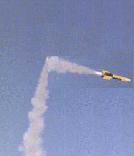What Could Possibly Go Wrong?

Good you asked. Two things will cause a difference between your calculation and where your rocket actually goes. First of all, the equations may be accurate, but ROCKETS ARE NOT! A rocket will vary in its performance for three main reasons:

• rocket motor thrust can vary by 10% either way - this can make a big difference
• the actual drag coefficient of your rocket depends a lot on how you built it - the shape of the fins and the nose, the launch lugs you used, the paint job and so on.
• your rocket's stability - if it flies funny it flies low.

Secondly, the equations make some approximations. I've minimized these as much as possible, as you will see. The three biggest approximations are

• constant air density - as you go up the air gets thinner, by about 10% for every 1,000 meters. So in other words this is not much of a concern at all up to about 3,000 feet, after that it starts to affect the accuracy. For model rockets don't worry about it, for high-power flights that go to five or six thousand feet (or more), get a rough estimate by using these equations with an average air density, and cross-check using a simulation.
• constant motor thrust - in reality the motor thrust varies during the burn, but we've used a constant average value. I've compared simulations using constant thrust and using the actual motor thrust curve and the effect on peak altitude calculations is negligible. The total impulse you use for the motor has a much bigger effect than varying the thrust.
• constant motor weight - in reality the motor mass changes because you're burning up the propellant. How you deal with the rocket mass is important for getting accurate results.

How to Handle Rocket Mass

In the example above I hand-waved the value I use for motor mass, but in reality I'm very deliberate about the value I choose. As your rocket burns fuel, the mass of the rocket changes. I estimate the mass of the rocket during the boost phase by adding

• the empty weight of the rocket,
• the weight of the empty motor casing, and
• half the weight of the propellant.

During the coast phase I use

• the empty weight of the rocket, and
• the weight of the empty motor casing,
• THAT'S IT, since the propellant's all burned up at this point.

The empty weight of the rocket is given to you as part of its specifications. You can also get the numbers on motor mass and propellant mass from the catalogs (the Estes or Aerotech catalog has their's, for instance) or you can estimate them as follows:

For fast estimation of high power (AP) motors in the field I have a cool cheat - the fully loaded motor mass in grams is roughly the same as the motor impulse in Newton-seconds. The propellant weight is roughly half the loaded motor weight. For Blackjacks add 100 grams.

For black powder (Estes) motor casings, I use a ballpark value of 0.5 ounce. I believe that's plenty good enough.

In general, to find the weight of the propellant I use a rocketry equation that states that the impulse of the motor in Newton-seconds is equal to the mass of the propellant multiplied by the exhaust velocity, or
I = M * Vex

Then the mass of the propellant, in kilograms, is the impulse divided by the exhaust velocity, for which 800 m/s is a good number for a common black-powder motor. (If you're going high power and using composites use Vex = 2000 m/s). So to get the mass of propellant just divide the impulse by 800, which gives it to you in kg. Multiply this number by 35.27 to get the propellant weight in ounces. Of course, the easy way is to get the numbers from the catalog.

Remember since you are burning the propellant up during the boost phase, use half the calculated propellant mass as an average value.Note: if you've done any reading on rocket physics, then just plugging in an average value for the rocket mass should seem like an awful idea. THE rocket equation is derived by calculating exactly the effect of this changing mass. We can get away with using a simplification because our model rockets only have a propellant mass of 10-40% of the payload weight. By contrast, the propellent for the space shuttle is 25 times the payload weight, and the changing mass cannot be approximated by an average value.

For typical model rockets, this approximation leads to an error of less than one percent, and allows us then to use an expression that accounts for wind resistance, a much more important factor. That in fact is the secret to the accuracy of these equations.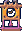How Long Will It Take To Get That High?

Oh, yeah, that's an easy calculation now. The time from burn-out to apogee (high point) we will call ta. To find it just calculate:

• qa = sqrt(M*g / k)
• qb = sqrt(g*k / M)
• ta = arctan(v / qa) / qb

So in our example above, where M = 0.05398, k = 0.000217 and v = 118 m/s (at burnout) then

• qa = sqrt(0.05398*9.8 / 0.000217) = 49.35
• qb = sqrt(9.8*0.000217 / 0.05398) = 0.1986
• ta = arctan(118 / 49.35) / 0.1986 = 5.915 seconds
Note: you should find arctan in radians.

This is the time from burnout to apogee and should correlate closely with the delay time on the ejection charge on your engine. For total time launch to apogee, add burn time t and ta. The burn time we found above was t = 1.5 seconds, so the total time in our example is
t + ta = 1.5 + 5.915 = 7.415 seconds.How Did You Figure This Out?

Since I posted the derivation of these equations I've received a very nice (much prettier) version in PDF format from Dr. Christian Strutz in Germany which you can get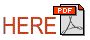Here is the no-frills (original) version:

Boost Phase: Velocity at Burnout

• Rocket thrust = T
• Force of gravity = M*g
• Drag force on rocket = 0.5*rho*Cd*A*v^2 = k*v^2
• Net force on rocket = F = T - M*g - k*v^2
• Newton's Second Law: F = M*a = M*(dv/dt) = T - M*g - kv^2
• Collecting terms: dt = M*dv / (T - M*g - k*v^2) = (M / k)*(dv / [q^2 - v^2])
where I've defined q = sqrt([T - M*g] / k)
• Integrating both sides (finite integral from 0 to v) and rearranging:
t = (M / k)*(1 / [2*q])*ln([q+v] / [q-v])

God this makes me wish I had something better than html for these equations.
Anyway,

• Simplifying a bit: 2*k*q*t / M = ln([q+v] / [q-v])
Set x = 2*k*q / M and then
• Solve for v:
v = q*[1 - exp(-x*t)] / [1 + exp(-x*t)]

Boost Phase: Altitude at Burnout

• Newton's Second Law Again
F = M*a = M*(dv/dt) = M*(dv/dy)*(dy/dt) = M*v*(dv/dy) = T - M*g - k*v^2
• Rearranging: dy = M*v*dv / (T - M*g - k*v^2)
• Integrating both sides (finite integral from 0 to v) and rearranging:
y = (-M / 2*k)*ln([T - M*g - k*v^2] / [T - M*g])

Coast Phase: Distance Travelled from Velocity v to Zero

• Newton's Second Law Yet Again
F = M*a = M*v*(dv/dy) = - M*g - k*v^2
• Rearranging: dy = M*v*dv / (- M*g - k*v^2)
• Integrating both sides: y = [+M / (2*k)]*ln([M*g + k*v^2] / [M*g])

Coast Phase: Time to Velocity Zero

• Newton's Second Law in the Time form
F = M*a = M*(dv/dt) = - M*g - k*v^2
• Rearranging: dt = M*dv / (- M*g - k*v^2)
• Integrating both sides: t = ([M/k]/sqrt[M*g/k])*arctan(v / sqrt[M*g/k])

Simplifies (some) to: t = sqrt(M / [k*g])*arctan(v / sqrt[M*g/k])

Approximation Using Static Rocket Mass

THE rocket equation assumes a dynamic mass m(t) = m0 - (dm/dt)*t, where dm/dt is a constant. When this expression is substituted into the above Second Law equations, they become intractable and must be solved with numerical methods. I therefore use a static expression for the mass M of the rocket. I will call the velocity found using the dynamic expression "vd", and the velocity found using the static expression "vs".

Define

• Vx = exhaust velocity, speed of propellant leaving rocket
• mr = mass of rocket, when EMPTY
• mp = mass of propellant (total)

Then we have

• THE rocket equation (dynamic mass): vd = Vx * ln([mr+mp] / mr)
• The "static rocket mass" equation: vs = Vx * (mp / mr)
• The static equation equivalent to my method of using average rocket mass is:
vs = Vx * (mp / [mr + 0.5*mp]).

Then a measure of the error induced by my method is E = 1 - vs / vd. Let's suppose the extreme case (for a model rocket) that the propellant is half the total weight of the rocket, or mp = mr = m. Then
E = 1 - vs / vd = 1 - [ (m / {m+0.5*m}) / ln({m+m} / m) ] = 1 - [1 / {ln(2) * 1.5}] = 0.04, or 4% error.

You can verify for yourself that for propellants that are somewhat smaller proportions of the rocket mass, the error is much smaller. The propellant has to exceed 67% of the total rocket mass before a 10% error is induced.How Stuff Works by Marshall Brain

Won't Someone Do All This Calculating For Me?

Sure. Just Push The Button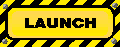The Rocket Simulator Free web based model rocket simulator

Or try this one (requires Flash)...University of New Mexico's Rocket Altitude Calculator Free web based model rocket simulator

Or geek out on this simple spreadsheet.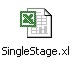Handy spreadsheet for altitude calculation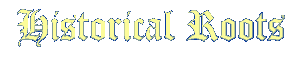I just recently heard from none other than Len Fehskens, who points out that in the 1960's he derived a form of the equations on this page. Shortly thereafter Doug Malewicki independently developed the same result, and the equations were named the "Fehskens-Malewicki equations". You can find references to these equations in Topics in Advanced Model Rocketry by G. K. Mandell, G. J. Caporaso, and W. P. Bengen, published in 1973 by the MIT Press, or in Estes TR-10 Altitude Prediction Charts, or you can go to Tom Beach's page on the subject. While the Fehskens-Malewicki equations are in a slightly different form, they are mathematically equivalent to the equations shown above, derived some 30 years later. Which just goes to demonstrate the old saying, "great minds think alike". Of course, there's another old saying, "idle hands are the devil's playthings". After working with the equations, you can be the judge as to which applies.

Questions

Your questions and comments regarding this page are welcome. You can e-mail Randy Culp (Tripoli #6926) for inquiries, suggestions, new ideas or just to chat.

And hey! Check out my certification level 1 rocket!Or... my level two certification rocket - a White Sands V2.My next project... a Warlok, bumblebee style...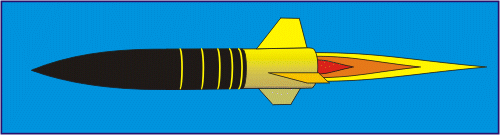Updated 04 August 2020